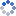加载中…用户1639993743
• 博客等级：• 博客积分：0
• 博客访问：589,953
• 关注人气：489
• 获赠金笔：0支
• 赠出金笔：0支
• 荣誉徽章：

## [原创]MATLAB中关于0.1-0.3+0.2不等于0的解释

(2012-10-12 18:47:43)

### 杂谈

[原创]MATLAB中关于0.1-0.3+0.2不等于0的解释

1. >> 0.1+0.2-0.3

2. ans =

3.    5.5511e-17

4. >> 0.1-0.3+0.2

5. ans =

6.    2.7756e-17

1. function [x_double,s,c,f]=ieee2double(x_ieee)
2. % 将IEEE编码转换为双精度数据
3. % x_double=(-1)^s*2^(c-1023)*(1+f)，双精度数据
4. % x_ieee，IEEE编码
5. % s，符号位，长度1
6. % c，指数位，长度11
7. % f，尾数位，长度52
8. %
9. s=bin2dec(x_ieee(1));
10. c=bin2dec(x_ieee(2:12));
11. m=bin2dec(x_ieee(13:64)');
12. % 为了保证精度，使用符号运算
13. f=sym('1/2').^(1:52)*m;
14. x_double=(-1)^s*2^(c-1023)*(1+f);

1. function [x_ieee,s,c,f]=double2ieee(x_double)
2. % 将双精度数据转换为IEEE编码
3. % x_double=(-1)^s*2^(c-1023)*(1+f)，双精度数据
4. % x_ieee，IEEE编码
5. % s，符号位，长度1
6. % c，指数位，长度11
7. % f，尾数位，长度52

8. if x_double>0
9.     s='0';
10. else
11.     s='1';
12. end
13. n=floor(log2(x_double));
14. c=dec2bin(n+1023,11);
15. f=dec2bin(round((x_double/2^n-1)*2^52),52);
16. x_ieee=[s,c,f];

1. >> x_double=0.1;
2. >> x_ieee_01=double2ieee(x_double)

3. x_ieee_01 =

4. 0011111110111001100110011001100110011001100110011001100110011010

1. IEEE(0011111110111001100110011001100110011001100110011001100110011001)
2. <<br style="word-wrap: break-word; ">
3. IEEE(0011111110111001100110011001100110011001100110011001100110011010)

1. >> x_double_01_left=ieee2double('0011111110111001100110011001100110011001100110011001100110011001')

2. x_double_01_left =

3. 7205759403792793/72057594037927936

4. >> double(x_double_01_left)-0.1 % 看到没有，第一个IEEE编码和0.1还是有差距的

5. ans =

6.   -1.3878e-17

7. >> x_double_01_right=ieee2double('0011111110111001100110011001100110011001100110011001100110011010')

8. x_double_01_right =

9. 3602879701896397/36028797018963968

10. >> double(x_double_01_right)-0.1 % 第二个IEEE编码和0.1就没有区别了，但是第二个IEEE编码也不是0.1的真实编码，而是距离最近的一个，换句话说0.1是没有准确的IEEE编码的，当然还有很多数据也没有准确的IEEE编码

11. ans =

12.      0

1. % 0.1的编码转换
2. >> x_ieee_01=double2ieee(0.1) % 0.1 IEEE编码

3. x_ieee_01 =

4. 0011111110111001100110011001100110011001100110011001100110011010

5. >> x_double_01=ieee2double(x_ieee_01)

6. x_double_01 =

7. 3602879701896397/36028797018963968

8. % 0.2的编码转换
9. >>  x_ieee_02=double2ieee(0.2) % 0.2 IEEE编码

10. x_ieee_02 =

11. 0011111111001001100110011001100110011001100110011001100110011010

12. >>  x_double_02=ieee2double(x_ieee_02)

13. x_double_02 =

14. 3602879701896397/18014398509481984

15. % 0.3的编码转换
16. >> x_ieee_03=double2ieee(0.3) % 0.3 IEEE编码

17. x_ieee_03 =

18. 0011111111010011001100110011001100110011001100110011001100110011

19. >> x_double_03=ieee2double(x_ieee_03)

20. x_double_03 =

21. 5404319552844595/18014398509481984

1. >> x_double_01-x_double_03+x_double_02

2. ans =

3. 1/36028797018963968

4. >> 1/36028797018963968

5. ans =

6.    2.7756e-17

7. >> 0.1-0.3+0.2

8. ans =

9.    2.7756e-17

0